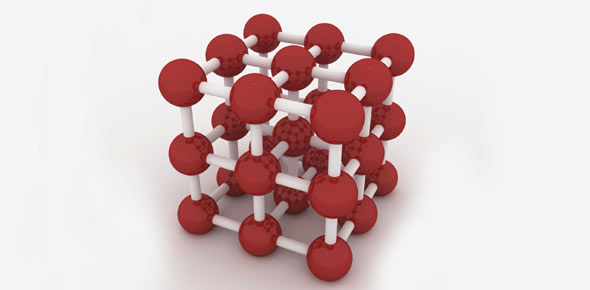# Semester Review: Units 1-5

48 Questions | Attempts: 171
ShareSettingsSemester Review over Units 1-5. Each question is labeled with what UNIT it came from so you can go back and study it if you have trouble with it.

• 1.
Which term describes the process of testing a hypothesis?
• A.

Creating a Hypothesis

• B.

Doing an Experiment

• C.

Drawing a Conclusion

• D.

Researching

• 2.
Unit 4:  Which of the following elements are transition metals? (You may select multiple answers)
• A.

Manganese (Mn)

• B.

Silicon (Si)

• C.

Strontium (Sr)

• D.

Gold (Au)

• 3.
Unit4:  Which element has the largest atomic radius?
• A.

Nitrogen (N)

• B.

Zinc (Zn)

• C.

Tungsten (W)

• D.

• 4.
Unit 4:  Order the following elements in order of increasing ionization energy: Hg, Fr, Cl, F, As
• A.

F, Cl, As, Hg, Fr

• B.

Fr, Hg, As, Cl, F

• C.

As, F, Cl, Fr, Hg

• D.

Hg, Fr, Cl, F, As

• 5.
Unit 4- Lithium (Li) is an alkaline earth metal.
• A.

True

• B.

False

• 6.
Unit 4:  Which of the following elements are Lanthanoids? (You may select multiple answers)
• A.

• B.

Einsteinium (Es)

• C.

Potassium (K)

• D.

Terbium (Tb)

• 7.
Unit 4:  Which element has a mass number of 32?
• A.

Sulfur (S)

• B.

Germanium (Ge)

• C.

Chlorine (Cl)

• D.

Arsenic (As)

• 8.
Unit 4:  How many neutrons are present in a Carbon-14 atom?
• A.

4 neutrons

• B.

6 neutrons

• C.

8 neutrons

• D.

10 neutrons

• 9.
Unit 4:  An atom of which element has 12 protons, 12 neutrons, and 12 electrons?
• A.

Carbon (C)

• B.

Nitrogen (N)

• C.

Magnesium (Mg)

• D.

Sodium (Na)

• 10.
Unit 4:  What is the name of an atom with an atomic mass number of 18 and 8 protons?
• A.

Argon-40

• B.

Oxygen-16

• C.

Oxygen-18

• D.

Fluorine-18

• 11.
Unit 4:  Calcium (Ca) always has 20 protons.
• A.

True

• B.

False

• 12.
Unit 4:  True or False: Na+ is an anion.
• A.

True

• B.

False

• 13.
Unit 5:  What is the name for the ion CO32- ?
• A.

Anion

• B.

Cation

• C.

Group of atoms

• D.

Molecule

• 14.
Unit 5:  Is OH- an element, ion, or compound?
• A.

Element

• B.

Ion

• C.

Compound

• 15.
Unit 3:  What term describes the process of a substance changing from solid to gas?
• A.

Condensation

• B.

Deposition

• C.

Sublimation

• D.

Evaporation

• 16.
Unit 3:  Which of the following is a chemical change?
• A.

Glass breaking

• B.

• C.

A towel absorbing water

• D.

A nail rusting in the rain

• 17.
Unit 3:  An example of intensive property is color.
• A.

True

• B.

False

• 18.
Unit 4:  What is the mass number of the element Tin (Sn)?
• A.

118.7

• B.

119

• C.

168.7

• D.

78.7

• 19.
Unit 4:  How many protons are present in an atom of Silver (Ag)?
• A.

108

• B.

94

• C.

61

• D.

47

• 20.
Unit 2:  What describes a substance that is firm where molecules can only vibrate in place?
• A.

Solid

• B.

Liquid

• C.

Gas

• 21.
Unit 2:  What describes a substance that can change shape but has a fixed volume and its molecules are loose?
• A.

Solid

• B.

Liquid

• C.

Gas

• 22.
Unit 3:  What describes a substance that has no definite shape or volume and its molecules are completely free?
• A.

Solid

• B.

Liquid

• C.

Gas

• 23.
Unit 4:  An neutron is negatively charged.
• A.

True

• B.

False

• 24.
Unit 4:  Which part(s) of an atom are located in the nucleus?
• A.

Proton

• B.

Neutron

• C.

Electron

• 25.
Unit 4:  What is the complete electron configuration for Chlorine (Cl)?
• A.

1p22p22s63p23s5

• B.

1s22s22p63s23p5

• C.

3p5

• D.

1s22s22p63s23p6

## Related TopicsBack to top
×

Wait!
Here's an interesting quiz for you.